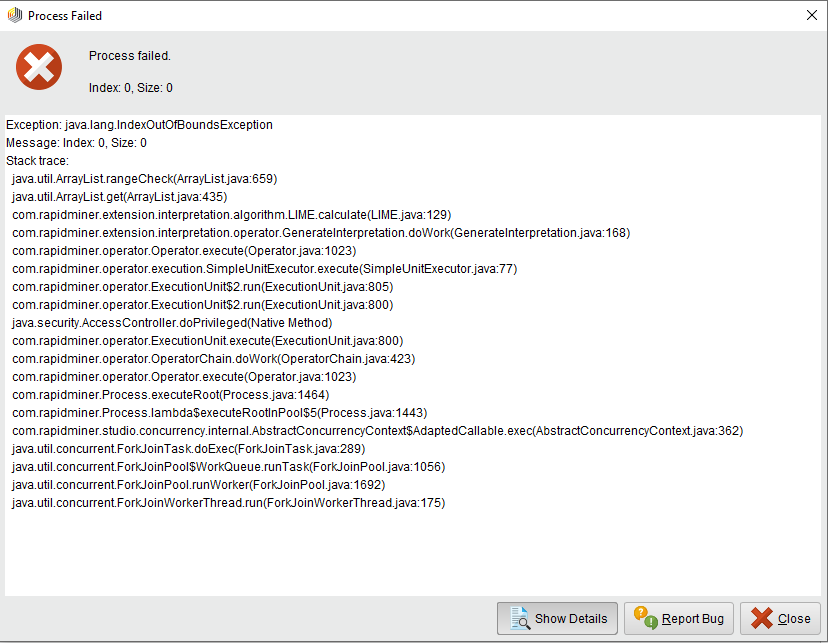# Exception occur when use Generate Interpretation operator with Python Learner

Member Posts: 1Contributor I
I am trying to use Generate Interpretation operator with Python learner but it keeps give me this Exception, I have tried several models in the Python code such as SVM and GausianMixture.Also the Log shows this warning "Custom Python Learner: The number of regular attributes of the given example set does not fit the number of attributes of the training example set, training: 7, application: 8". Maybe this warnig and IndexOutOfBoundsException are related.

The Apply Model operator works well with the Python Learner, also I am sure that the number of attributes are all the same, and I tried to remove the label to see if there is a difference, but the same error apears when using Generate Interpetation.

What could be the problem is?

Tagged:

• Administrator, Moderator, Employee, RapidMiner Certified Analyst, RapidMiner Certified Expert, University Professor Posts: 3,321RM Data Scientist

the line failing is the line were we try to find the confidence column after scoring. Does your python script provide confidences or only predictions?

Can you maybe provide an example for it? This is either a bug or we need a proper error message here.

Cheers,
Martin
- Head of Data Science Services at RapidMiner -
Dortmund, Germany
• Administrator, Moderator, Employee, RapidMiner Certified Analyst, RapidMiner Certified Expert, University Professor Posts: 3,321RM Data Scientist
Hi,
just ran this one here and it worked just fine:
`<?xml version="1.0" encoding="UTF-8"?><process version="9.10.001"><br>  <context><br>    <input/><br>    <output/><br>    <macros/><br>  </context><br>  <operator activated="true" class="process" compatibility="9.10.001" expanded="true" name="Process" origin="GENERATED_TUTORIAL"><br>    <parameter key="logverbosity" value="init"/><br>    <parameter key="random_seed" value="2001"/><br>    <parameter key="send_mail" value="never"/><br>    <parameter key="notification_email" value=""/><br>    <parameter key="process_duration_for_mail" value="30"/><br>    <parameter key="encoding" value="SYSTEM"/><br>    <process expanded="true"><br>      <operator activated="true" class="retrieve" compatibility="9.10.001" expanded="true" height="68" name="Retrieve Titanic Training" width="90" x="45" y="34"><br>        <parameter key="repository_entry" value="//Samples/data/Titanic Training"/><br>      </operator><br>      <operator activated="true" class="nominal_to_numerical" compatibility="9.10.001" expanded="true" height="103" name="Nominal to Numerical" width="90" x="179" y="34"><br>        <parameter key="return_preprocessing_model" value="false"/><br>        <parameter key="create_view" value="false"/><br>        <parameter key="attribute_filter_type" value="all"/><br>        <parameter key="attribute" value=""/><br>        <parameter key="attributes" value=""/><br>        <parameter key="use_except_expression" value="false"/><br>        <parameter key="value_type" value="nominal"/><br>        <parameter key="use_value_type_exception" value="false"/><br>        <parameter key="except_value_type" value="file_path"/><br>        <parameter key="block_type" value="single_value"/><br>        <parameter key="use_block_type_exception" value="false"/><br>        <parameter key="except_block_type" value="single_value"/><br>        <parameter key="invert_selection" value="false"/><br>        <parameter key="include_special_attributes" value="false"/><br>        <parameter key="coding_type" value="dummy coding"/><br>        <parameter key="use_comparison_groups" value="false"/><br>        <list key="comparison_groups"/><br>        <parameter key="unexpected_value_handling" value="all 0 and warning"/><br>        <parameter key="use_underscore_in_name" value="false"/><br>      </operator><br>      <operator activated="true" class="python_scripting:python_learner" compatibility="9.10.001" expanded="true" height="82" name="Python Learner" width="90" x="313" y="34"><br>        <parameter key="editable" value="true"/><br>        <parameter key="operator" value="{&#10;  &quot;name&quot;: &quot;Custom Python Learner&quot;,&#10;  &quot;dropSpecial&quot;: true,&#10;  &quot;capabilities&quot;: [&quot;numerical attributes&quot;, &quot;binominal label&quot;, &quot;polynominal label&quot;],&#10;  &quot;parameters&quot;: [&#10;    {&#10;      &quot;name&quot;: &quot;1st_parameter&quot;,&#10;      &quot;description&quot;: &quot;By default parameters are of type string\.&quot;,&#10;      &quot;optional&quot;: true&#10;    },&#10;    {&#10;      &quot;name&quot;: &quot;2nd_parameter&quot;,&#10;      &quot;description&quot;: &quot;This is an example of an mandatory integer parameter with a default value 100\.&quot;,&#10;      &quot;type&quot;: &quot;integer&quot;,&#10;      &quot;optional&quot;: false,&#10;      &quot;value&quot;: 100&#10;    },&#10;    {&#10;      &quot;name&quot;: &quot;3rd_parameter&quot;,&#10;      &quot;description&quot;: &quot;An example of a categorical parameter type\.&quot;,&#10;      &quot;type&quot;: &quot;category&quot;,&#10;      &quot;categories&quot;: [&quot;Category A&quot;, &quot;Category B&quot;, &quot;Category C&quot;, &quot;Default Category&quot;],&#10;      &quot;value&quot;: &quot;Default Category&quot;&#10;    }&#10;  ]&#10;}.from pandas import DataFrame&#10;from sklearn\.naive_bayes import GaussianNB&#10;&#10;# Mandatory training function\. When implementing a supervised learner, the&#10;# input data will be split into the feature vector X and the label vector y\.&#10;# Parameters are passed in as plain Python dictionary\.&#10;def rm_train(X, y, parameters):&#10;&#9;# This example does not make use of the parameter to configure the model\.&#10;&#9;# However, printing the dictionary will show its values in the log\.&#10;&#9;print(parameters)&#10;&#9;# You can return any Python object as model\. Sci-kit learn classifiers such&#10;&#9;# as the Gaussian Naive Bayes are just one example\.&#10;&#9;clf = GaussianNB()&#10;&#9;model = clf\.fit(X, y)&#10;&#9;return model&#10;&#10;# Mandatory application function\. The input data set X is guaranteed to have&#10;# have the same columns and column order as seen during training\. The model is&#10;# the of the same type as the model return during training\.&#10;def rm_apply(X, model):&#10;&#9;prediction = DataFrame(model\.predict(X))&#10;&#9;probabilities = DataFrame(model\.predict_proba(X))&#10;&#9;probabilities\.columns = model\.classes_&#10;&#9;# The first return value must be a Pandas DataFrame with a single column&#10;&#9;# and of the size as X\. The second return value is optional and can be used&#10;&#9;# to return probabilities (if any)\.&#10;&#9;return prediction, probabilities&#10;"/><br>        <parameter key="use_default_python" value="true"/><br>        <parameter key="package_manager" value="conda (anaconda)"/><br>        <parameter key="2nd_parameter" value="100"/><br>        <parameter key="3rd_parameter" value="Default Category"/><br>      </operator><br>      <operator activated="true" class="multiply" compatibility="9.10.001" expanded="true" height="103" name="Multiply" width="90" x="447" y="55"/><br>      <operator activated="true" class="filter_example_range" compatibility="9.10.001" expanded="true" height="82" name="Filter Example Range" width="90" x="581" y="85"><br>        <parameter key="first_example" value="1"/><br>        <parameter key="last_example" value="5"/><br>        <parameter key="invert_filter" value="false"/><br>      </operator><br>      <operator activated="true" class="interpretation:generate_interpretation" compatibility="0.4.001" expanded="true" height="124" name="Generate Interpretation" width="90" x="715" y="34"><br>        <parameter key="algorithm" value="LIME"/><br>        <parameter key="sample_size" value="100"/><br>        <parameter key="redraw_local_samples" value="true"/><br>        <parameter key="explanation_algorithm" value="Correlation"/><br>        <parameter key="locality" value="0.2"/><br>        <parameter key="maximal_explaining_attributes" value="3"/><br>        <parameter key="use_local_random_seed" value="false"/><br>        <parameter key="local_random_seed" value="1992"/><br>      </operator><br>      <connect from_op="Retrieve Titanic Training" from_port="output" to_op="Nominal to Numerical" to_port="example set input"/><br>      <connect from_op="Nominal to Numerical" from_port="example set output" to_op="Python Learner" to_port="training set"/><br>      <connect from_op="Python Learner" from_port="model" to_op="Generate Interpretation" to_port="mod"/><br>      <connect from_op="Python Learner" from_port="example set" to_op="Multiply" to_port="input"/><br>      <connect from_op="Multiply" from_port="output 1" to_op="Generate Interpretation" to_port="training"/><br>      <connect from_op="Multiply" from_port="output 2" to_op="Filter Example Range" to_port="example set input"/><br>      <connect from_op="Filter Example Range" from_port="example set output" to_op="Generate Interpretation" to_port="test"/><br>      <connect from_op="Generate Interpretation" from_port="example set" to_port="result 1"/><br>      <portSpacing port="source_input 1" spacing="0"/><br>      <portSpacing port="sink_result 1" spacing="0"/><br>      <portSpacing port="sink_result 2" spacing="0"/><br>      <description align="left" color="yellow" colored="false" height="64" resized="false" width="289" x="90" y="148">This tutorial process requires the package<br>                                Scikit-Learn.<br>                            </description><br>    </process><br>  </operator><br></process><br><br>`

- Head of Data Science Services at RapidMiner -
Dortmund, Germany